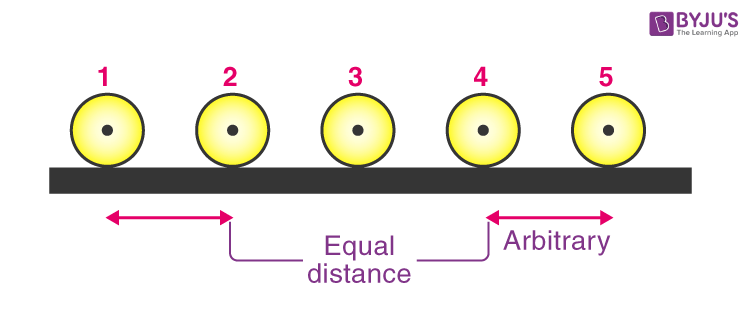# Motion in a Straight Line

## What Is Motion in a Straight Line?

If an object changes its position with respect to its surroundings with time, then it is called in motion. It is a change in the position of an object over time. Motion in a straight line is nothing but linear motion. As the name suggests, it’s in a particular straight line, thus it can be said that it uses only one dimension.

### Types of Linear Motion

The linear motion, also called the Rectilinear Motion can be of two types:

1. Uniform linear motion with constant velocity or zero acceleration
2. Non-Uniform linear motion with variable velocity or non-zero acceleration

Linear motion is the most straightforward kind of one-dimensional motion. As Newton’s first law of motion suggests, an object will either be at rest or continue to move in a straight line with a uniform velocity unless and until an external force is applied to it.

You are suggested not to confuse linear motion with general motion. As we discussed, linear motion is a one-dimensional motion. Still, in general, the motion has magnitude and direction both, i.e. an object’s position and velocity are described in vector quantities.

Read More: Vector and Scalar Quantities

### Uniform Motion in a Straight Line

If a body travels in a straight line and covers an equal amount of distance in an equal interval of time, it is said to have uniform motion. In simple words, a body is said to have uniform acceleration if the rate of change of its velocity remains constant.The ball covers equal distance in equal intervals of time

Example:

If a car travels at a speed of 60 km/hour, it will cover a 1 km/minute. In this sense, the motion of car acceleration is uniform.

## Watch the video and learn about motion in one dimension### Non-uniform Motion in a Straight Line

Unlike the uniform acceleration, the body is said to have a non-uniform motion when the velocity of a body changes by unequal amounts in equal intervals of time—the rate of change of its velocity changes at different points of time during its movement.

Example:

A boy is kicking a football. It might cover 4 meters in the first attempt, 6 meters in the second change, 9 meters in the third attempt and so on as per the velocity exerted by the boy.

## The below video provides the Top 10 NTSE Important Questions on Motion Class 9## Motion in a Straight Line Formulas

Following are the formulas of motion in a straight line:

 v =u + at $$\begin{array}{l}s=ut+\frac{1}{2}at^{2}\end{array}$$ v2 = u2 + 2as

You may want to check out the following related videos for better understanding of motion and its characteristics.

#### Motion#### Motion in One Dimension (1D)